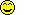## Confuse-a-Cat [General Sta­tis­tics]

Hi ElMaestro,

» I am sorry that I am once again not able to tell what I am looking for.

No, I’m sorry that I’m not able to comprehend your message. As you know, walnut-sized brain.

» I am not talking about simulations and not about transformations either.

OK.

» Two functions, generally, and the key word is really generally, when are they (or their results) to be considered independent?

I think:
• The results of f(x) and g(x) should have a very (very!) low correlation.
• Both should convey different information about the properties of x.
• What else?

» We can think of f=mean(x) and g=median(x). I guess we can easily do a mental picture of plotting means versus medians, often seeing the strong relationship. Visually appealing.

Go back to my script and

med.spl <- aggregate(xs[, 2], list(sample = xs$sample), median) plot(mean.spl$x, med.spl$x) cor(mean.spl$x, med.spl\$x)

» Independence?

No. Both are measures of the location of the data. IMHO, that would not be suitable to construct a test as stated at the end of this post.

» OK then let us say f=range(x) and g=sine function of the range (x).» Or an F statistics with a variance in both f=numerator and g=denominator in an unbalanced anova.

Oh dear!

» Or Cmax and AUCt (which I guess are correlated and dependent(?), …

Correlated, yes. Highly sometimes. In some cases not so much (recall that one). Why? Duno. Though correlation  causation.
Actually there are hidden (confounding) variables – the entire PK stuff – which drives the apparent correlation. So are they dependent even with a high correlation? I would say no. Both depend on the underlying PK.

» … but the example is not great in my perspective since the two functions are not applied to a random sample but to a time series).

Yep.

» There is no end to the possible examples.

» Without debating to much about the specific cases, how do we generally approach it to define two (outcomes of) functions as being independent?

See above. Maybe I’m completely wrong.

» Which mathematical/algebraic/statistical/whatever properties of functions render them mutually independent? When I understand it, I think or hope I will understand the nature of independence.
» For inspiration: Are estimates of any two statistical moments independent? If yes, why? Is it only the first and second? Why? Is it generally so? Why? Etc. I am looking for the general clarity.

Sorry, again.

Dif-tor heh smusma 🖖Helmut SchützThe quality of responses received is directly proportional to the quality of the question asked. 🚮
Science QuotesIng. Helmut Schütz